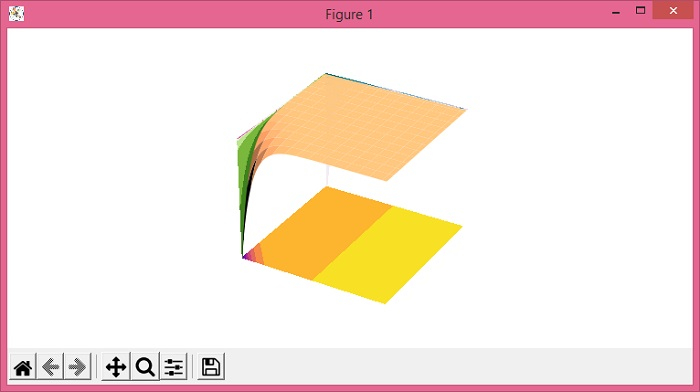# Plot Matplotlib 3D plot_surface with contour plot projection

To plot 3d plot_surface with contour plot projection, we can use plot_surface() and contourf() methods.

## Steps

• Set the figure size and adjust the padding between and around the subplots.

• Create x, y, X, Y and Z data points using numpy.

• Create a new figure or activate an existing figure using figure() method.

• Add an '~.axes.Axes' to the figure as part of a subplot arrangement, with 3D projection.

• Use plot_surface() method to create a surface plot.

• Create a 3D filled contour plotm using contourf() method.

• Trurn off the axes.

• To display the figure, use show() method.

## Example

import numpy as np
from matplotlib import pyplot as plt

plt.rcParams["figure.figsize"] = [7.50, 3.50]
plt.rcParams["figure.autolayout"] = True

x = np.arange(-5, 5, 0.1)
y = np.arange(-5, 5, 0.1)
X, Y = np.meshgrid(x, y)
Z = X * np.exp(-X - Y)

fig = plt.figure()

surf = ax.plot_surface(X, Y, Z, rstride=8, cstride=8, alpha=1, cmap="copper")
ax.contourf(X, Y, Z, zdir='z', offset=np.min(Z), cmap="plasma")
ax.contourf(X, Y, Z, zdir='x', offset=-5, cmap="PiYG_r")
ax.contourf(X, Y, Z, zdir='y', offset=5, cmap="PuBuGn")

ax.axis('off')
plt.show()

## Output